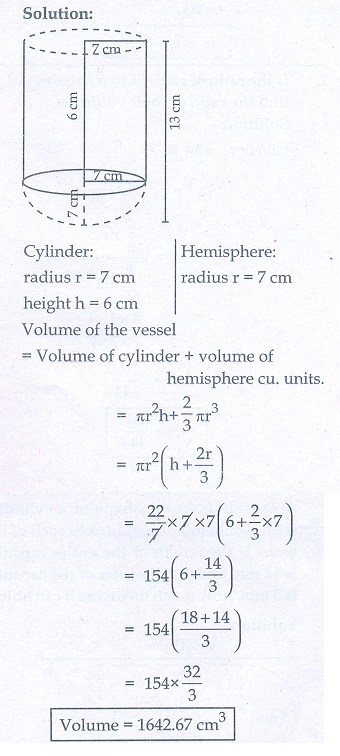Home | | Maths 10th Std | Exercise 7.3: Volume and Surface Area of Combined Solids

# Exercise 7.3: Volume and Surface Area of Combined Solids

Maths Book back answers and solution for Exercise questions - Mathematics : Mensuration: Volume and Surface Area of Combined Solids: Exercise Problem Questions with Answer

### Exercise 7.3

1. A vessel is in the form of a hemispherical bowl mounted by a hollow cylinder. The diameter is 14 cm and the height of the vessel is 13 cm. Find the capacity of the vessel.2. Nathan, an engineering student was asked to make a model shaped like a cylinder with two cones attached at its two ends. The diameter of the model is 3 cm and its length is 12 cm. If each cone has a height of 2 cm, find the volume of the model that Nathan made.3. From a solid cylinder whose height is 2.4 cm and the diameter 1.4 cm, a cone of the same height and same diameter is carved out. Find the volume of the remaining solid to the nearest cm3.4. A solid consisting of a right circular cone of height 12 cm and radius cm standing on a hemisphere of radius 6 cm is placed upright in a right circular cylinder full of water such that it touches the bottom.Find the volume of the water displaced out of the cylinder, if the radius of the cylinder is 6 cm and height is 18 cm.5. A capsule is in the shape of a cylinder with two hemisphere stuck to each of its ends. If the length of the entire capsule is 12 mm and the diameter of the capsule is 3 mm, how much medicine it can hold?6. As shown in figure a cubical block of side 7 cm is surmounted by a hemisphere. Find the surface area of the solid.7. A right circular cylinder just enclose a sphere of radius r units. Calculate

(i) the surface area of the sphere

(ii) the curved surface area of the cylinder

(iii) the ratio of the areas obtained in8. A shuttle cock used for playing badminton has the shape of a frustum of a cone is mounted on a hemisphere. The diameters of the frustum are 5 cm and 2 cm. The height of the entire shuttle cock is 7 cm. Find its external surface area.1. 1642.67 cm3

2. 66 cm3

3. 2.46 cm3

4. 905.14 cm3

5. 56.51 cm3

6. 332.5 cm2

7.(i) 4πr2pr sq. units (ii) 4πr2pr sq. units (iii) 1:1

8. 73.39 cm2

Tags : Problem Questions with Answer, Solution | Mensuration | Mathematics , 10th Mathematics : UNIT 7 : Mensuration
Study Material, Lecturing Notes, Assignment, Reference, Wiki description explanation, brief detail
10th Mathematics : UNIT 7 : Mensuration : Exercise 7.3: Volume and Surface Area of Combined Solids | Problem Questions with Answer, Solution | Mensuration | Mathematics